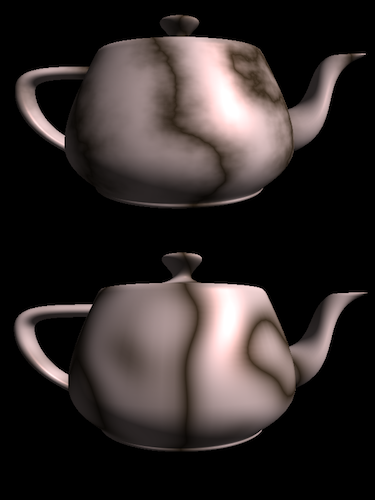«

### 2010

A number of months back, I wrote about how to use OpenGL shaders in Clojure, using Penumbra. This introduced an s-expression representation of GLSL, which allowed for an idiomatic specification of shader code. It didn’t, however, cut down on the boilerplate that surrounds the OpenGL programmable pipeline.

There are two steps in the programmable pipeline: the vertex shader and the fragment shader. The vertex shader transforms geometry from the world-space to screen-space. The geometry is then discretized into pixels, and then each pixel is processed through the fragment shader. For a more in-depth look at this process, check out this set of articles.

This is referred to as a pipeline because data flows from the vertices to the vertex shader to the fragment shader. Since these shaders are meant to be swappable, the vertex shader has to declare what data it passes the fragment shader, and the fragment shader has to declare what data it receives from the vertex shader. Mismatched declarations are allowed, which can be useful, but mostly it’s just redundant and increases the potential for errors. If we’re willing to treat the pipeline as a single entity, our specification can become shorter, simpler, and much more straightforward to develop.

So, let’s revisit the marble teapot:

In the previous example, we used the Perlin noise lookup that’s built into GLSL. This approach had a lot of limitations; this built-in function only works properly on a fraction of all hardware, and it can only be used within the vertex shader. As a result, the marble coloration was per-vertex rather than per-pixel, and looked a lot less detailed.To create our own per-pixel Perlin noise implementation, we need a block of 3-D random noise. We can get this by creating a 3-D texture, which is seeded with random numbers from -1 to 1. To perform a per-pixel lookup, each pixel needs to know its location in the world space. This is different than its location with respect to the camera; if we used the location in camera-space, our teapot looks like this:

We will also need to know the normal on a per-pixel basis, to allow for per-pixel lighting. These two values, along with the transformed screen-space location, are represented as a hash-map at the end of the vertex shader. These values are effectively the “return value” of the vertex shader.

``````(defpipeline marble

:vertex {position (float3 :vertex)
normal (normalize
(float3
(* :normal-matrix
:normal)))
:position (* :model-view-projection-matrix
:vertex)}

:fragment (let [noise 0
scale 0.5
pos (* position
(float3 (/ 1 (.x (dim %)))))]
(dotimes [i octaves]
(+= noise (* (% pos) scale))
(*= scale 0.5)
(*= pos (float3 2)))
(let [marble (-> position .x
(+ noise) (* 2)
sin abs)
mixed (mix [0.2 0.15 0.1 1]
[0.8 0.7 0.7 1]
(pow marble 0.75))]
(* mixed (lighting 0 normal)))))
``````

Notice that in the fragment code, we reference both position and normal, which are defined in the vertex shader. The `%` variable represents the 3-D texture that’s seeded with random values. Also notice that while this looks a lot like Clojure code, it uses operators like `+=` and `*=` that belie the underlying C implementation. The above code expands to this, and the complete code can be found here.

Stay tuned for future announcements. I hope to have vertex buffers implemented in the next week or two.